Updated: 12/11/2021

# Enzyme Kinetics

0%
Topic
Review Topic
0
0
N/A
N/A
Questions
3
0
0
0%
0%
Evidence
2
0
0
0%
0%
Videos / Pods
1
Topic
Images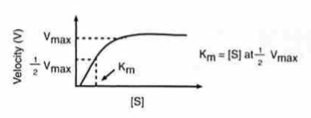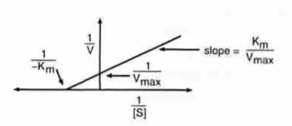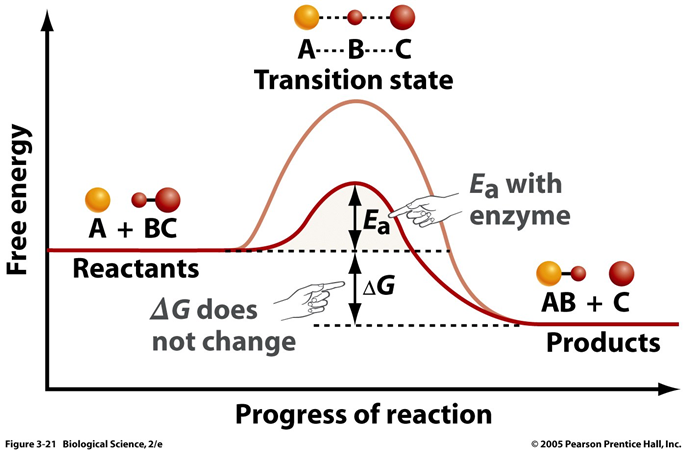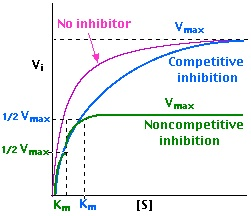• Overview
• for two molecules to react
• they must be within bond-forming distance
• possess enough kinetic energy to overcome the activation energy (Ea)
• factors that affect these two conditions will either decrease or increase the reaction rate
• temperature: causes an increase in kinetic energy
• concentration of reactants: increases probability of collisions
• Gibbs free energy change (ΔG)
• is the free energy change between the products and the reactants
• reflects the direction of a reaction and amount of reactants and products at equilibrium, but does NOT determine the rates of reaction
• ΔG < 0: reaction is spontaneous and favors product formation
• ΔG = 0: reaction is at equilibrium and proceeds in both direction at equal rates
• ΔG > 0: reaction is nonspontaneous and favors reactant formation
• Ea determines the rate of the reaction
• a large Ea will have a slower rate
• a small Ea will have a faster rate
• enzymes lower the Ea allowing the reaction to proceed at a faster rate
• enzymes do NOT change the ΔG of the reaction just the Ea
• enzymes are sensitive to temperature and pH
• Enzymes Kinetics
• Michaelis-Menten Equation
•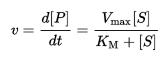• an equation that relates the initial reaction velocity (Vi) to the substrate concentration
• Vmax is directly proportional to the [E]
• Km is the Michaelis-Menten constant which represents the substrate concentration at which Vi is half the maximum velocity (Vmax)
• Km = [S] at 1/2 Vmax
• Km is related to the enzyme's affinity for the substrate [S]
• ↑ Km = ↓ affinity
• ↓ Km = ↑ affinity
• inhibitors affect these enzyme parameters
• competitive increases Km
• noncompetitive decreases Vmax
• Lineweaver-Burk Equation
• an inverted form of the Michaelis-Menten equation
• used to calculate Vmax and Km from experimental data at below enzyme saturation levels
• the equation is in the format y = ax + b (a is the slope and b is the y intercept)
• y = 1/Vi
• x = 1/[S]
• a = Km/Vmax
• b = 1/Vmax
• the smaller value of -1/Km, the greater the Km
• ↑ y-intercept = ↓ Vmax

You have never rated this topic.

Thank you. You can rate this topic again in 12 months.

Flashcards (0)
Cards
1 of 0
Questions (3)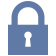Sorry, this question is forSorry, this question is for

(M1.PH.14.23) In your peripheral tissues and lungs, carbonic anhydrase works to control the equilibrium between carbon dioxide and carbonic acid in order to maintain proper blood pH. Through which mechanism does carbonic anhydrase exert its influence on reaction kinetics?

QID: 100088

Lowers the activation energy

67%

(278/413)

Changes the delta G of the reaction

8%

(34/413)

Raises the activation energy

8%

(32/413)

Lowers the free energy of products

5%

(21/413)

Lowers the free energy of reactants

7%

(28/413)

M 1 E

Select Answer to see Preferred Response

Evidence (2)
VIDEOS & PODCASTS (2)